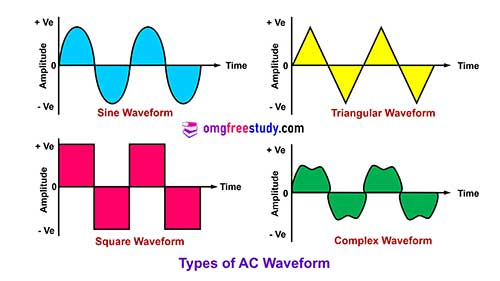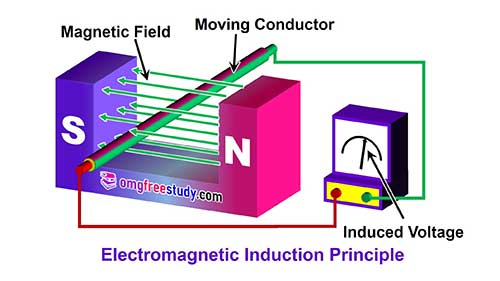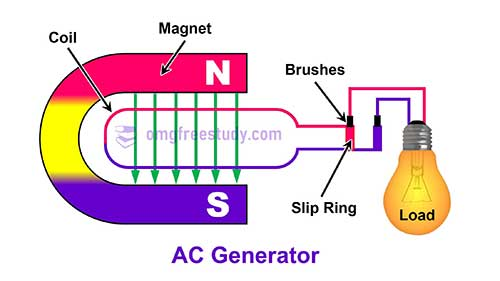# What is Alternating Current (AC)?

## Introduction

An alternating current (AC) in a circuit is one which the direction as well as amplitude of the current flow through it is change at regular intervals. In this type of circuit the current is supplied from an AC voltage source. The reversal of circuit current flow due to the polarity of an AC source changes at regular intervals.

Alternating current usually changes in its both value and direction. The current increases from zero to some maximum/ peak value, and then it drops back to zero, when it flows in one direction. Then this same pattern is repeated as it flows in opposite direction. The type of AC voltage source can be determined by the waveform in which the current is increases and decreases in particular manner. Different type of waveforms is shown in figure.## Generation of Alternating current

An Alternating current is used where a large amount of electrical power is required. Almost all of the electrical energy supplied for domestic, commercial and industrial purposes is alternating current (AC).

AC voltage is most preferable because it is much easier to transmit over a long distances, it’s cheaper to generate and power loss is low in it.

Generally AC equipment is more economical to maintain and less requirement of space per unit of power rating than the DC equipment.

Alternating current can be generated at higher voltages than DC, with less problems of heating and arcing. Some standard voltage values are 1.1 KV, 2.2 KV, 3.3 KV for low capacity and 6.6 KV (6600V), 11 KV (11000V) and 33 KV (33000V) for high capacity requirements.

The values of generated voltage are increased to 66 KV (66000V), 110 KV (110000), 220 KV (220000), 400 KV (400000) volts for transmission over long distances.

After the transmission end, the voltage is decreased for working values of 240V and 415V as per requirement.

## Basic Principle of AC Generator

The basic method of obtaining AC is by the use of an AC generator called as alternator. An AC generator is a machine that uses magnetism generator principle. Simply it is state that a voltage is induced in a conductor when the conductor is moved in a magnetic field, and conductor cut the lines of magnetic force. Then in a magnetic field around a conductor tends to set electrons in motion. The figure shows the basic generator principle.When we move the conductor through the magnetic field, a force is exerted on each of the free electrons within the conductor by the magnetic field. These forces add together and developed that voltage or induced into the conductor.

A voltage to be induced at the ends of the wire. This voltage changes in magnitude and polarity as the loop coil is rotated within the magnetic field. The direction of induced current can be find by Flemings Right hand rule.The force required to turn the loop coil can be obtained from various sources like steam turbines or by the water force.

The AC voltage induced in the coils is connected to a set of slip rings from which the external circuit receives the voltage through a set of brushes. An electromagnet is used to produce a stronger magnetic field. In this way we can produce Alternating Current (AC).

## MCQ Questions PDF of Module 6: AC Circuits

• Alternating current – terms & definitions – vector diagrams.
• Series resonance circuit.
• R-L, R-C and R-L-C parallel circuits.
• Parallel resonance circuits.
• Power, energy and power factor in AC single phase system – Problems.
• Power factor – Improvement of power factor.
• 3-Phase AC fundamentals